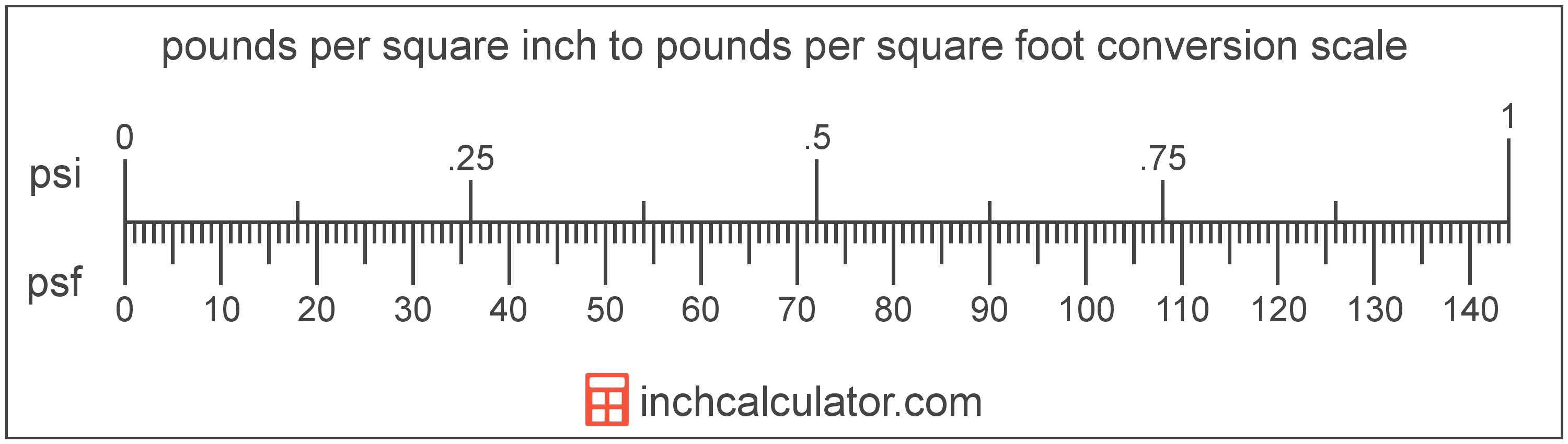# Pounds per Square Foot to Pounds per Square Inch Converter

Enter the pressure in pounds per square foot below to get the value converted to pounds per square inch.

Results in Pounds per Square Inch:1 psf = 0.006944 psi

Do you want to convert pounds per square inch to pounds per square foot?

## How to Convert Pounds per Square Foot to Pounds per Square Inch

To convert a measurement in pounds per square foot to a measurement in pounds per square inch, divide the pressure by the following conversion ratio: 144 pounds per square foot/pound per square inch.

Since one pound per square inch is equal to 144 pounds per square foot, you can use this simple formula to convert:

pounds per square inch = pounds per square foot ÷ 144

The pressure in pounds per square inch is equal to the pressure in pounds per square foot divided by 144.

For example, here's how to convert 500 pounds per square foot to pounds per square inch using the formula above.
pounds per square inch = (500 psf ÷ 144) = 3.472222 psiPounds per square foot and pounds per square inch are both units used to measure pressure. Keep reading to learn more about each unit of measure.

## What Are Pounds per Square Foot?

One pound per square foot is the pressure of equal to one pound-force per square foot.

The pound per square foot is a US customary and imperial unit of pressure. Pounds per square foot can be abbreviated as psf; for example, 1 pound per square foot can be written as 1 psf.

PSF can be expressed using the formula:
1 psf = 1 lbf / ft2

Pressure in pounds per square foot are equal to the pound-force divided by the area in square feet.

## What Are Pounds per Square Inch?

One pound per square inch is the pressure of equal to one pound-force per square inch.

The pound per square inch is a US customary and imperial unit of pressure. A pound per square inch is sometimes also referred to as a pound-force per square inch. Pounds per square inch can be abbreviated as psi; for example, 1 pound per square inch can be written as 1 psi.

PSI can be expressed using the formula:
1 psi = 1 lbf / in2

Pressure in pounds per square inch are equal to the pound-force divided by the area in square inches.

## Pound per Square Foot to Pound per Square Inch Conversion Table

Table showing various pound per square foot measurements converted to pounds per square inch.
Pounds Per Square Foot Pounds Per Square Inch
1 psf 0.006944 psi
2 psf 0.013889 psi
3 psf 0.020833 psi
4 psf 0.027778 psi
5 psf 0.034722 psi
6 psf 0.041667 psi
7 psf 0.048611 psi
8 psf 0.055556 psi
9 psf 0.0625 psi
10 psf 0.069444 psi
20 psf 0.138889 psi
30 psf 0.208333 psi
40 psf 0.277778 psi
50 psf 0.347222 psi
60 psf 0.416667 psi
70 psf 0.486111 psi
80 psf 0.555556 psi
90 psf 0.625 psi
100 psf 0.694444 psi
200 psf 1.3889 psi
300 psf 2.0833 psi
400 psf 2.7778 psi
500 psf 3.4722 psi
600 psf 4.1667 psi
700 psf 4.8611 psi
800 psf 5.5556 psi
900 psf 6.25 psi
1,000 psf 6.9444 psi